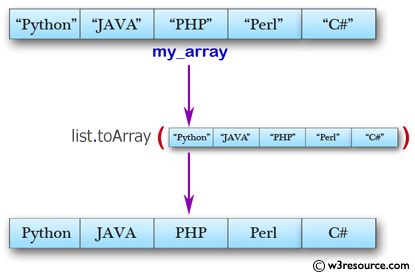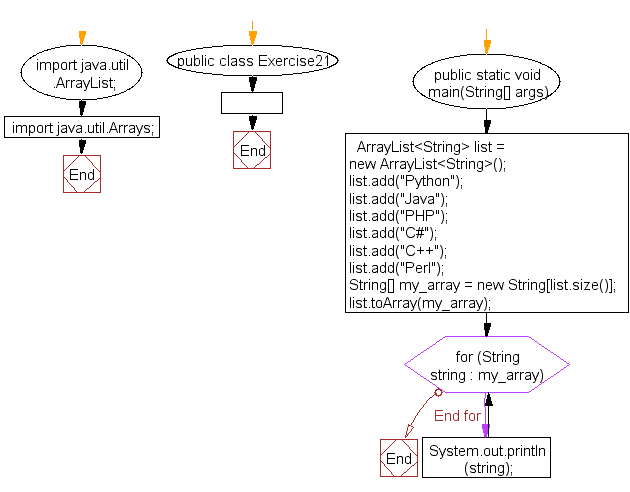﻿ Java exercises: Convert an ArrayList to an array - w3resource# Java Array Exercises: Convert an ArrayList to an array

## Java Array: Exercise-21 with Solution

Write a Java program to convert an ArrayList to an array.

Pictorial Presentation:Sample Solution:

Java Code :

``````import java.util.ArrayList;
import java.util.Arrays;
public class Exercise21 {
public static void  main(String[] args)
{
ArrayList<String> list = new ArrayList<String>();

String[]  my_array = new String[list.size()];

list.toArray(my_array);

for (String  string : my_array)
{
System.out.println(string);
}
}
}
```
```

Sample Output:

```Python
Java
PHP
C#
C++
Perl
```

Flowchart:Visualize Java code execution (Python Tutor):

Java Code Editor:

Improve this sample solution and post your code through Disqus

What is the difficulty level of this exercise?

Test your Programming skills with w3resource's quiz.

﻿

## Java: Tips of the Day

countOccurrences

Counts the occurrences of a value in an array.

Use Arrays.stream().filter().count() to count total number of values that equals the specified value.

```public static long countOccurrences(int[] numbers, int value) {
return Arrays.stream(numbers)
.filter(number -> number == value)
.count();
}
```

Ref: https://bit.ly/3kCAgLb